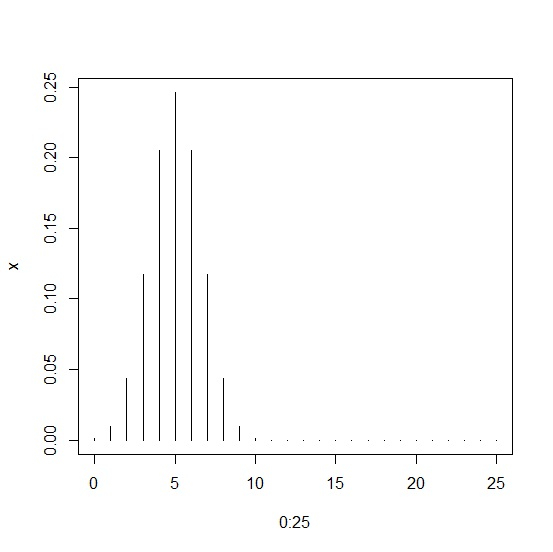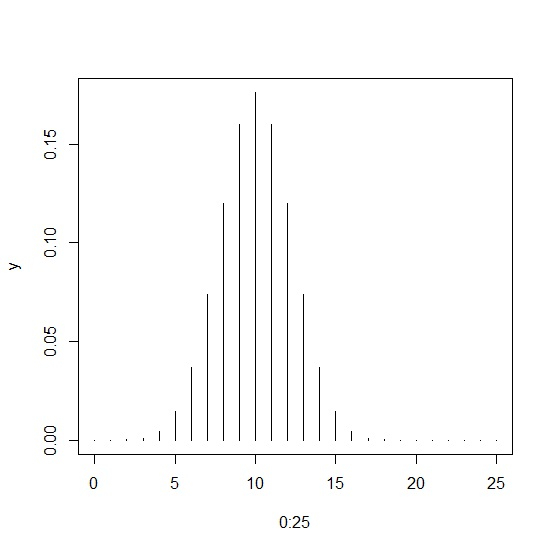# How to create a plot of binomial distribution in R?

A binomial distribution is based on the distribution of success and failure, the other two parameters of binomial distribution are the sample size and the probability of success. To create a plot of binomial distribution, we first need to define the density of the binomial distribution using dbinom function. The plotting can be done by using plot function with success and the density as shown in the below examples.

## Example

x<-dbinom(0:25,size=10,prob=0.5)
plot(0:25,x,type="h")

## Output## Example

y<-dbinom(0:25,size=20,prob=0.5)
plot(0:25,y,type="h")

## Output